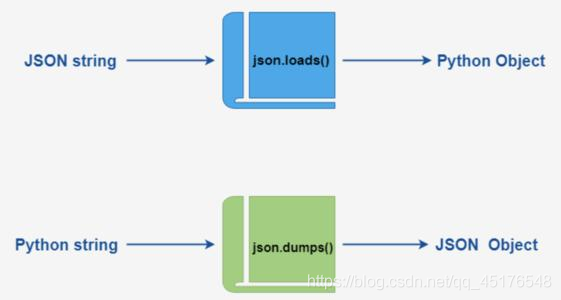### Python解析JSON对象的全过程记录

[导读] Python解析JSON对象的全过程记录...

### 前言

json处理模块的主要任务，是将一个JSON对象，转换成Python数据类型数据进行处理，或者反之，将Python数据类型数据，转换成JSON对象（字符串流），在不同的模块或者系统间传输。

### 1. JSON数据格式特点

1. 对象表示为键值对
2. 数据由逗号分隔
3. 花括号保存对象
4. 方括号保存数组
```{
"students": [
{ "name":"北山啦" , "age":20 },
{ "name":"张三" , "age":30 },
{ "name":"里斯" , "age":17 }
]
}
```
```{'students': [{'name': '北山啦', 'age': 20},
{'name': '张三', 'age': 30},
{'name': '里斯', 'age': 17}]}
```

JSON也支持各种数据类型，它的数据类型和Python各种数据类型之间的对比如下：

• object ―― dict
• array ―― list
• string ―― str
• number ―― int/float
• true/false ―― True/False
• null ―― None

### 2. 常用方法总结

• loads()：从JSON字符串中读取数据并转换成Python数据类型
• load()：从JSON文件中读取数据并转换成Python数据类型
• dumps()：将Python数据类型数据转换成JSON字符串
• dump():将Python数据类型数据转换成JSON字符串写入到文件

### 3. 系列化和反系列化3.1 系列化

```import json

data = {
"students": [
{ "name":"北山啦" , "age":20 },
{ "name":"张三" , "age":30 },
{ "name":"里斯" , "age":17 }
]
}
print(type(data))
print(data)
json_str = json.dumps(data)
print(type(json_str))
```
```
{'students': [{'name': '北山啦', 'age': 20}, {'name': '张三', 'age': 30}, {'name': '里斯', 'age': 17}]}

```

```import json

data = {
"students": [
{ "name":"北山啦" , "age":20 },
{ "name":"张三" , "age":30 },
{ "name":"里斯" , "age":17 }
]
}

print(type(data))
print(data)
json_str = json.dumps(data, separators=('>>','::'), indent=2)
print(json_str)
```
```
{'students': [{'name': '北山啦', 'age': 20}, {'name': '张三', 'age': 30}, {'name': '里斯', 'age': 17}]}
{
"students"::[
{
"name"::"\u5317\u5c71\u5566">>
"age"::20
}>>
{
"name"::"\u5f20\u4e09">>
"age"::30
}>>
{
"name"::"\u91cc\u65af">>
"age"::17
}
]
}
```

```import json

data = {
"students": [
{ "name":"北山啦" , "age":20 },
{ "name":"张三" , "age":30 },
{ "name":"里斯" , "age":17 }
]
}
with open("students.txt","w") as fp:
json.dump(data, fp, ensure_ascii=False)
print("finish")
```

finish

3.2 反系列化

```import json
with open("students.txt") as fp:
data = json.load(fp)
"""取出字典key为students的数据，
得到一个list，再从这个list中取第一个数据"""
print(data['students'])
```

{'name': '北山啦', 'age': 20}

parse_int参数

parse_int参数，这里我们简单将其指定为float类型。

```import json
with open("students.txt") as fp:
data = json.load(fp, parse_int = float)
print(data)
```

{'students': [{'name': '北山啦', 'age': 20.0}, {'name': '张三', 'age': 30.0}, {'name': '里斯', 'age': 17.0}]}

object_hook

```def fromJSON(dct): # 这里会对所有的字典数据类型都进行遍历
if isinstance(dct, dict) and 'students' in dct:
return dct['students']
else:
return Student(dct['name'], dct['age'])

import json

with open("students.txt") as fp:
data = json.load(fp, object_hook=fromJSON)
print(data)

```

[姓名: 北山啦, 年龄: 20, 姓名: 张三, 年龄: 30, 姓名: 里斯, 年龄: 17]

### 总结